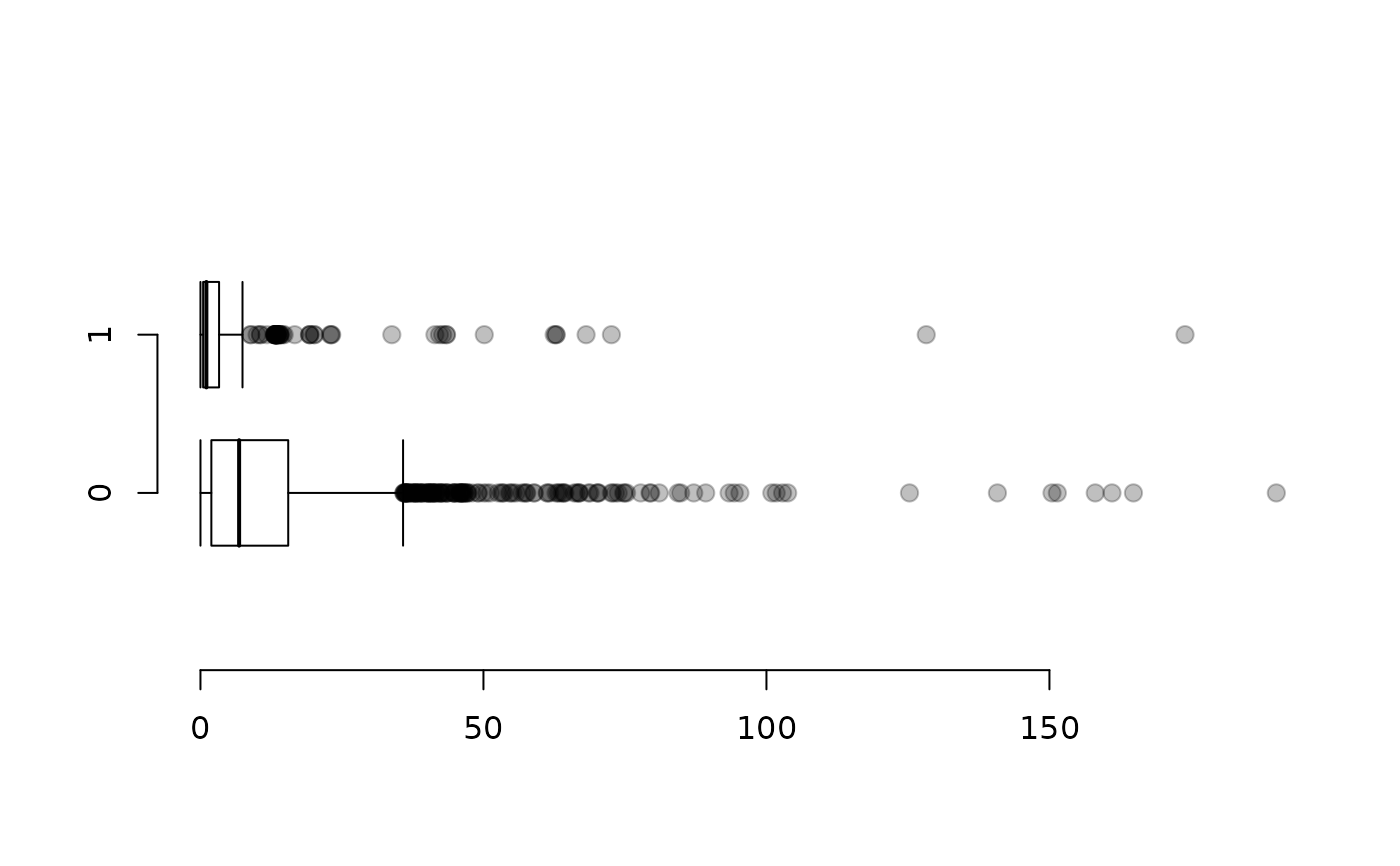An alternative to boxplot. Equations are not accepted. Instead, the second argument, fact, is used to split the data.

## Usage

boxPlot(
x,
fact = NULL,
horiz = FALSE,
width = 2/3,
lwd = 1,
lcol = "black",
medianLwd = 2,
pch = 20,
pchCex = 1.8,
col = grDevices::rgb(0, 0, 0, 0.25),
key = NULL,
axes = TRUE,
xlab = "",
ylab = "",
xlim = NULL,
ylim = NULL,
na.rm = TRUE,
...
)

## Arguments

x

A numerical vector.

fact

A character or factor vector defining the grouping for side-by-side box plots.

horiz

If TRUE, the box plot is oriented horizontally.

width

The width of the boxes in the plot. Value between 0 and 1.

lwd

Width of lines used in box and whiskers.

lcol

Color of the box, median, and whiskers.

medianLwd

Width of the line marking the median.

pch

Plotting character of outliers.

pchCex

Size of outlier character.

col

Color of outliers.

If FALSE, a new plot is created. Otherwise, the boxplots are added to the current plot for values of TRUE or a numerical vector specifying the locations of the boxes.

key

The order in which to display the side-by-side boxplots. If locations are specified in add, then the elements of add will correspond to the elements of key.

axes

Whether to plot the axes.

xlab

Label for the x axis.

ylab

Label for the y axis.

xlim

Limits for the x axis.

ylim

Limits for the y axis.

na.rm

Indicate whether NA values should be removed.

...

histPlot, dotPlot, densityPlot

David Diez

## Examples


# univariarate
boxPlot(email$num_char, ylab = "Number of characters in emails")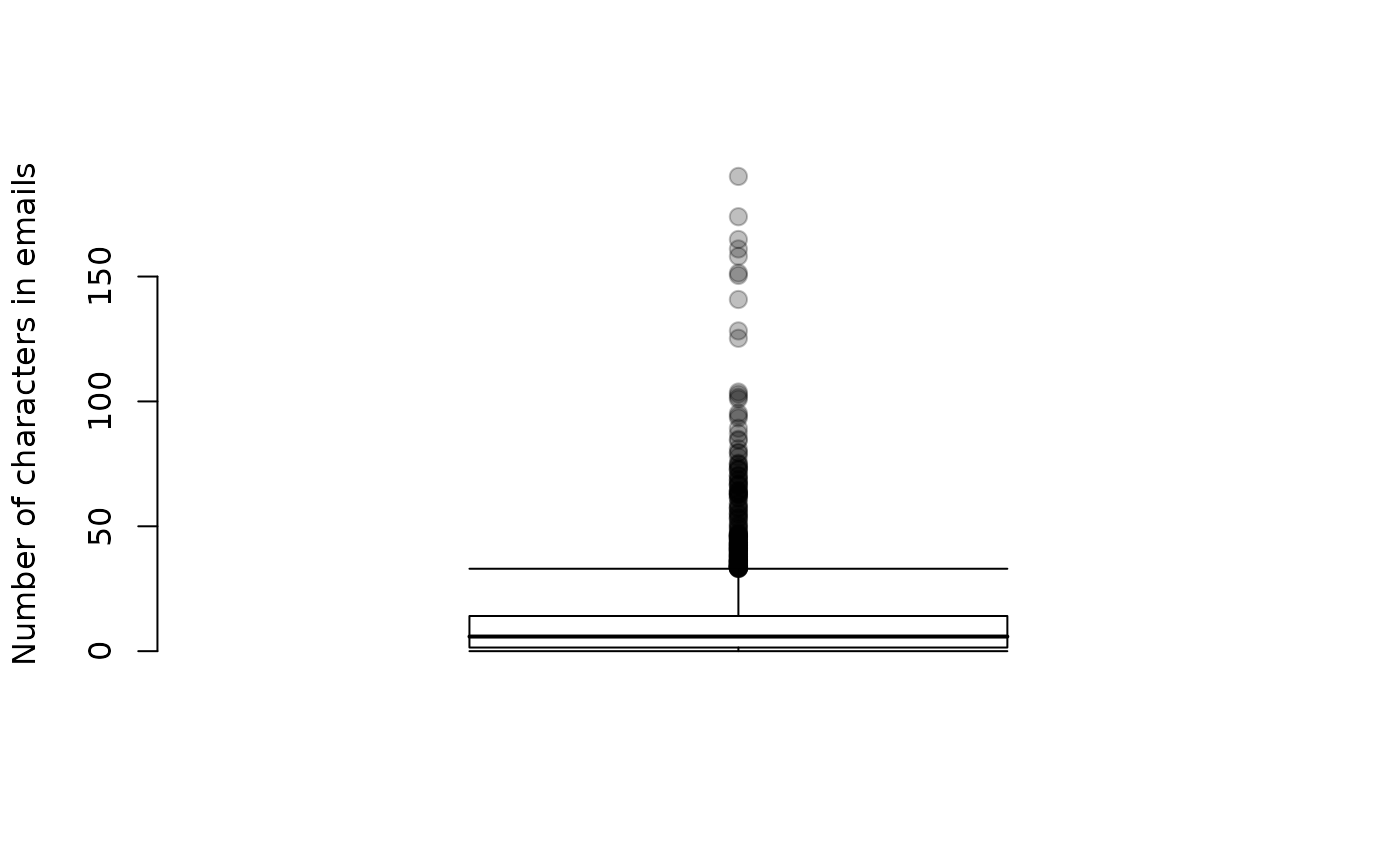# bivariate boxPlot(email$num_char, email$spam, xlab = "Spam", ylab = "Number of characters in emails" )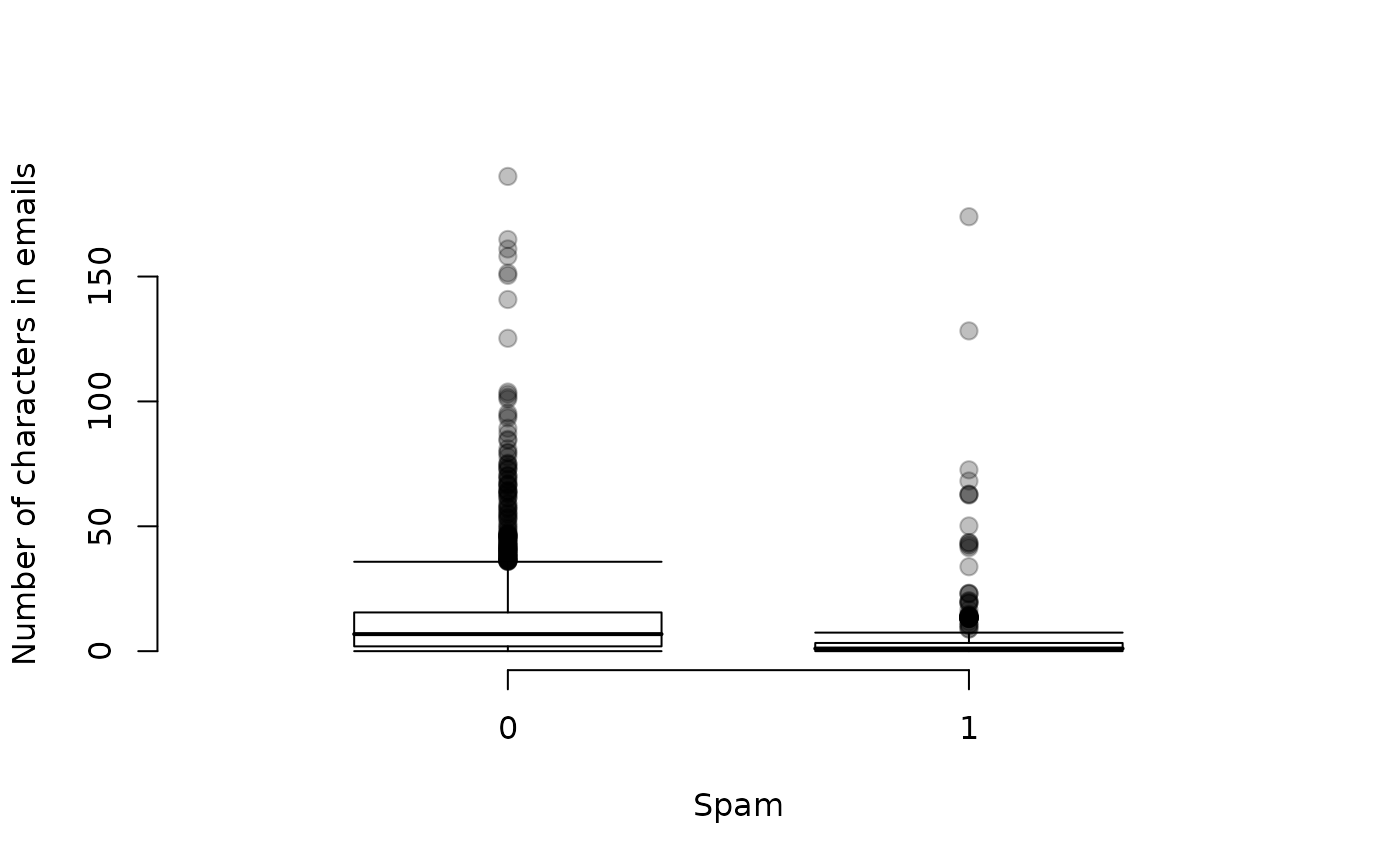# faded outliers boxPlot(email$num_char, email$spam, xlab = "Spam", ylab = "Number of characters in emails", col = fadeColor("black", 18) )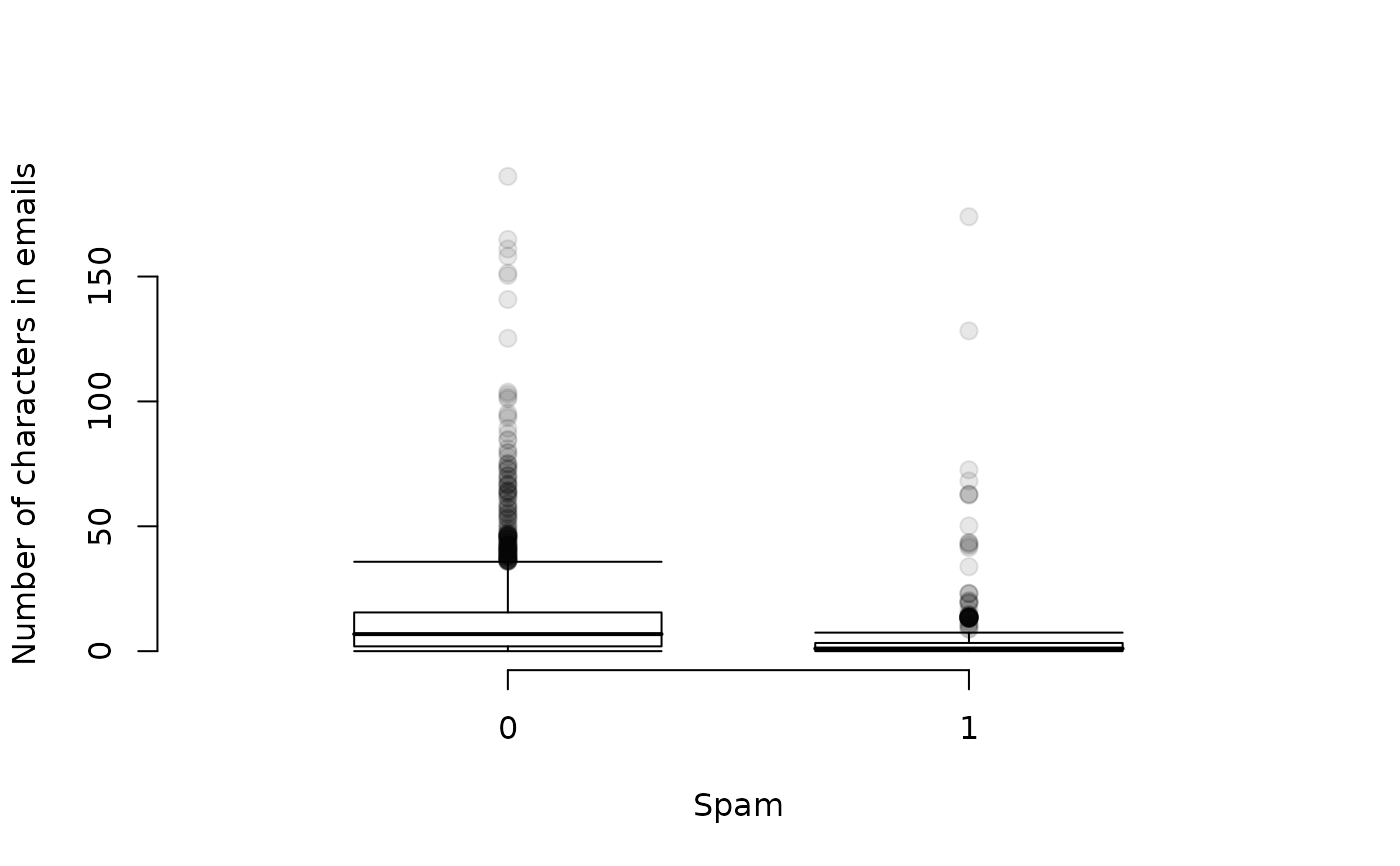# horizontal plots boxPlot(email$num_char, email$spam, horiz = TRUE, xlab = "Spam", ylab = "Number of characters in emails", col = fadeColor("black", 18) )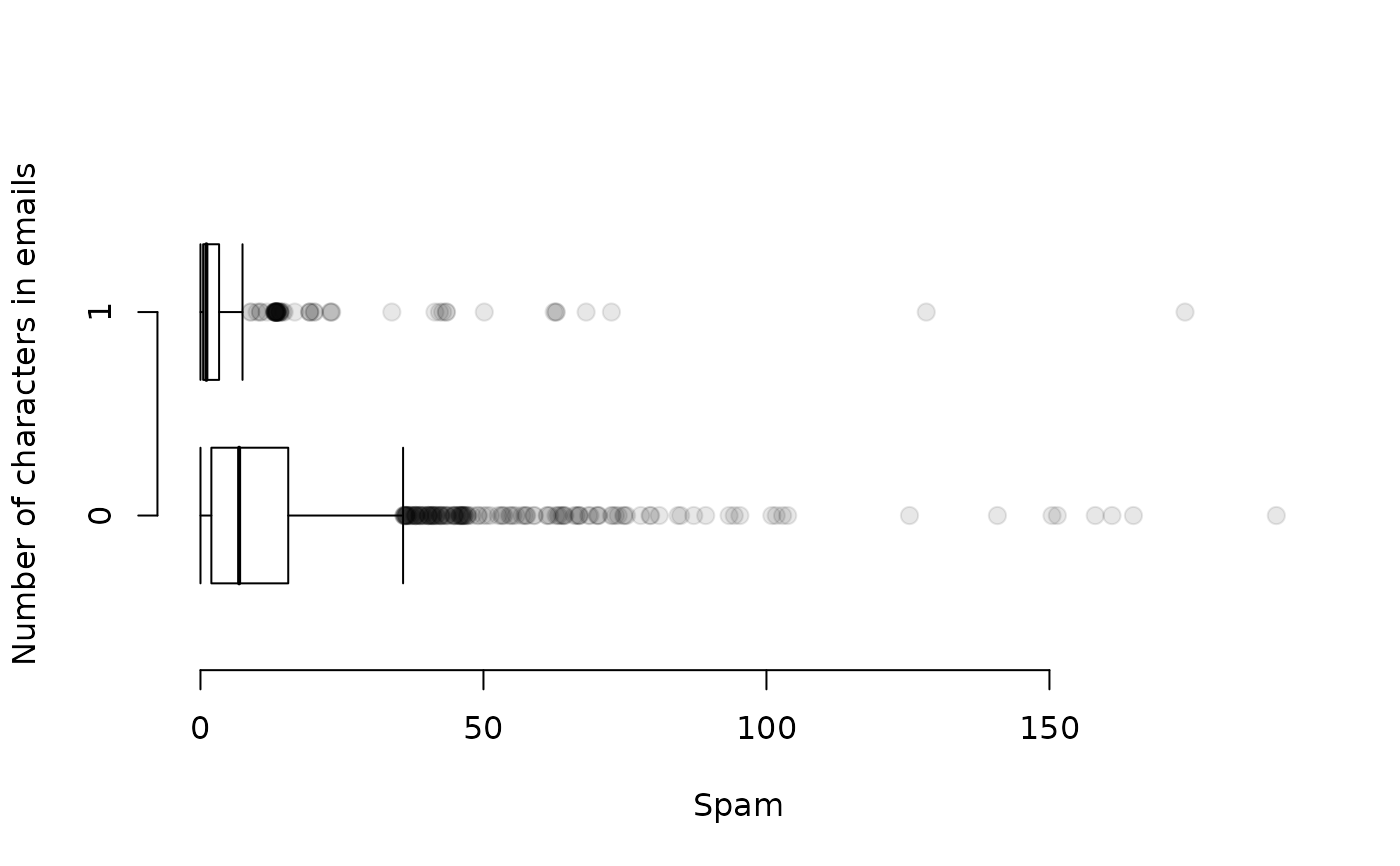# bivariate relationships where categorical data have more than 2 levels boxPlot(email$num_char, email$image, horiz = TRUE, xlab = "Number of attached images", ylab = "Number of characters in emails", col = fadeColor("black", 18) )# key can be used to restrict to only the desired groups boxPlot(email$num_char, email$image, horiz = TRUE, key = c(0, 1, 2), xlab = "Number of attached images (limited to 0, 1, 2)", ylab = "Number of characters in emails", col = fadeColor("black", 18) )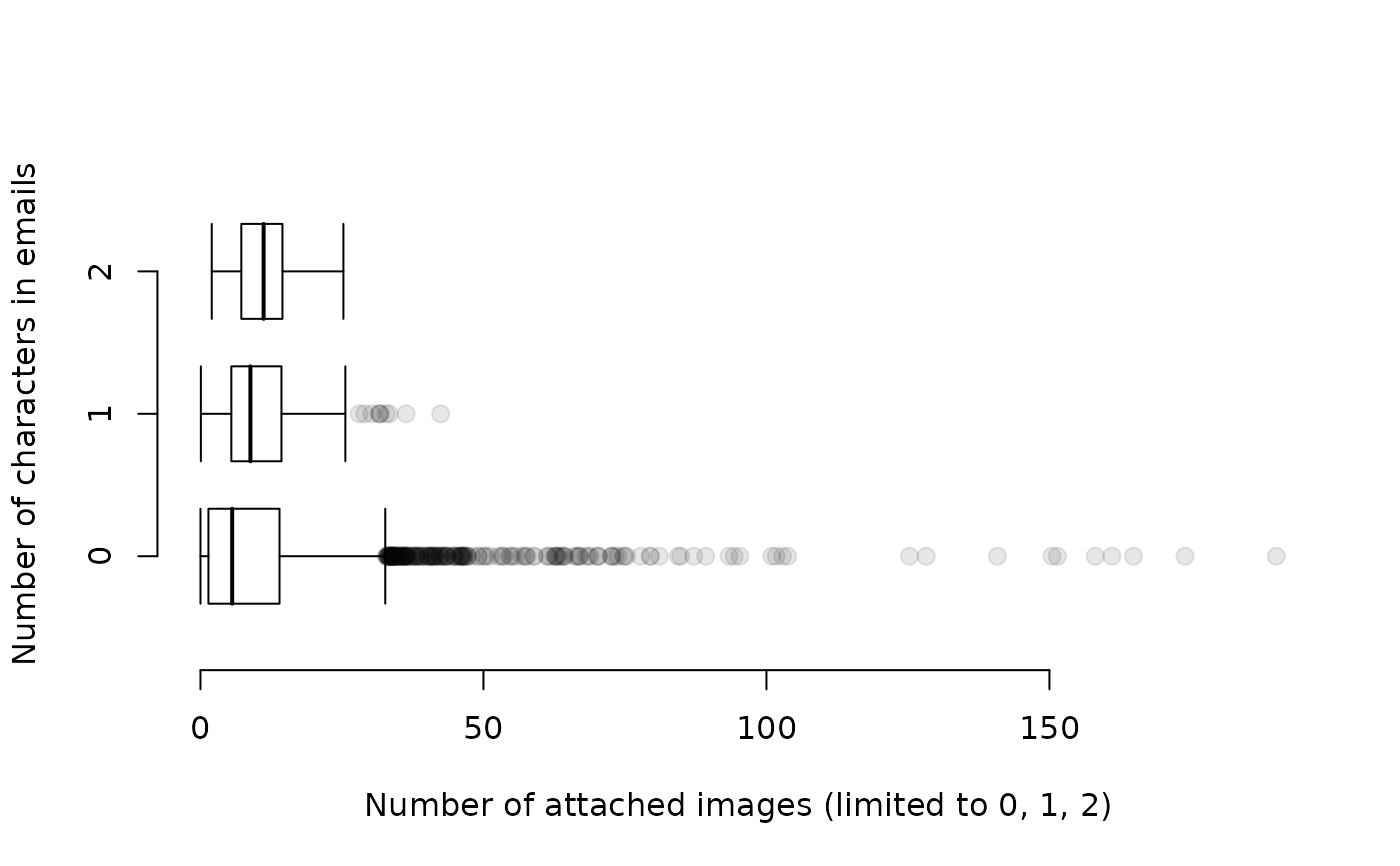# combine boxPlot and dotPlot boxPlot(tips$tip, tips$day, horiz = TRUE, key = c("Tuesday", "Friday") ) dotPlot(tips$tip, tips$day, add = TRUE, at = 1:2 + 0.05, key = c("Tuesday", "Friday") )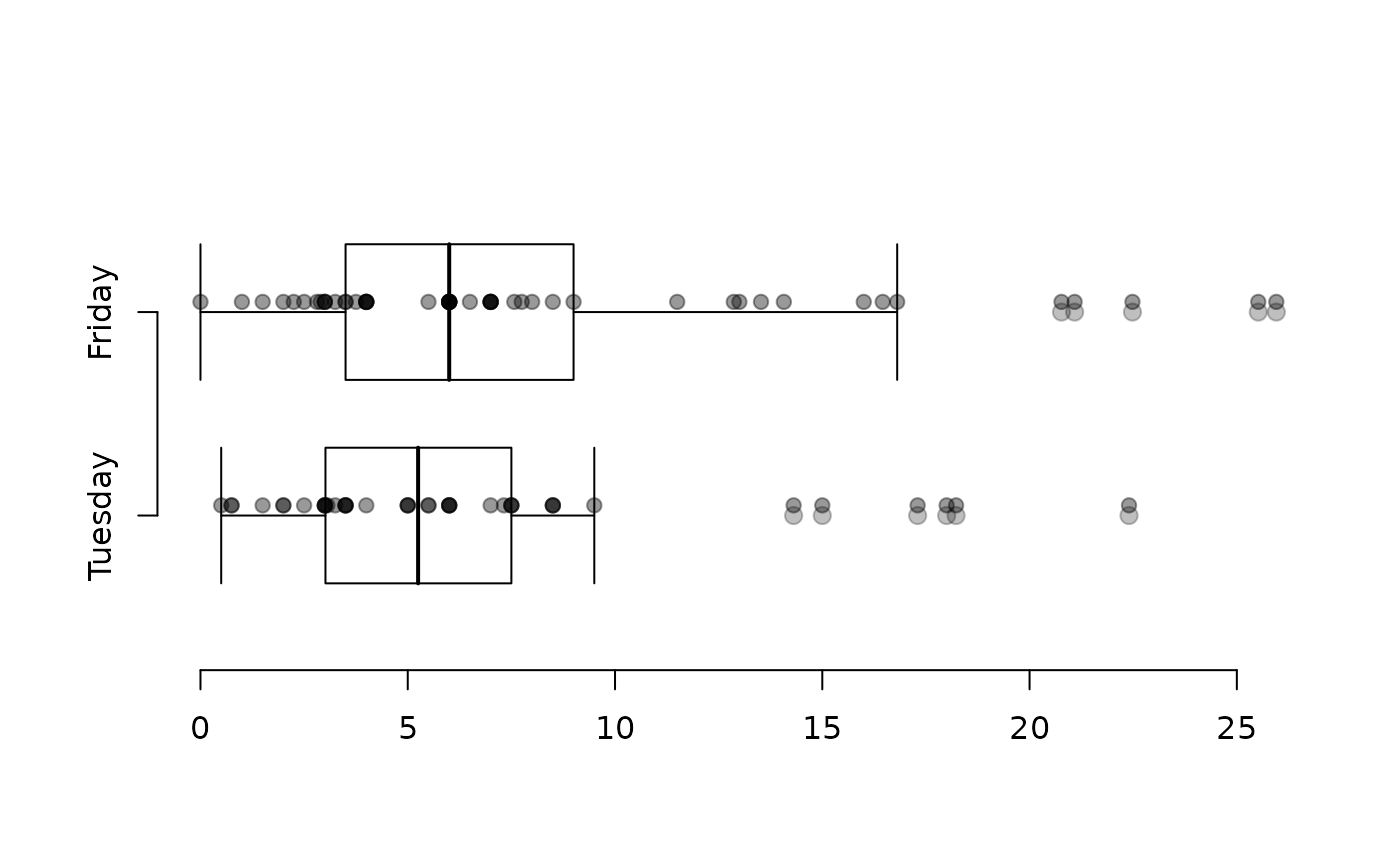# adding a box boxPlot(email$num_char[email$spam == 0], xlim = c(0, 3)) boxPlot(email$num_char[email$spam == 1], add = 2, axes = FALSE) axis(1, at = 1:2, labels = c(0, 1))boxPlot(email$num_char[email$spam == 0], ylim = c(0, 3), horiz = TRUE) boxPlot(email$num_char[email\$spam == 1], add = 2, horiz = TRUE, axes = FALSE)
axis(2, at = 1:2, labels = c(0, 1))